Next: HOW FAST FOURIER TRANSFORM Up: TWO-DIMENSIONAL FT Previous: Signs in Fourier transforms

## Examples of 2-D FT

An example of a two-dimensional Fourier transform of a pulse is shown in Figure 10.ft2dofpulse
Figure 9
A broadened pulse (left) and the real part of its FT (right).

Notice the location of the pulse. It is closer to the time axis than the frequency axis. This will affect the real part of the FT in a certain way (see exercises). Notice the broadening of the pulse. It was an impulse smoothed over time (vertically) by convolution with (1,1) and over space (horizontally) with (1,4,6,4,1). This will affect the real part of the FT in another way.

Another example of a two-dimensional Fourier transform is given in Figure 11. This example simulates an impulsive air wave originating at a point on the x-axis. We see a wave propagating in each direction from the location of the source of the wave. In Fourier space there are also two lines, one for each wave. Notice that there are other lines which do not go through the origin; these lines are called spatial aliases.'' Each actually goes through the origin of another square plane that is not shown, but which we can imagine alongside the one shown. These other planes are periodic replicas of the one shown.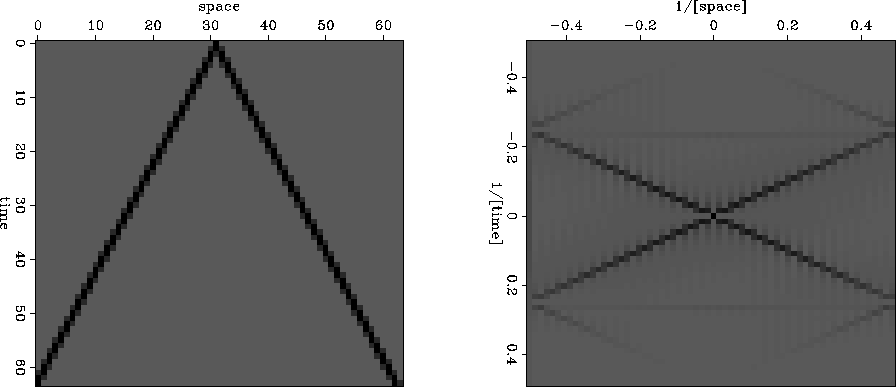airwave
Figure 10
A simulated air wave (left) and the amplitude of its FT (right).

## EXERCISES:

1. Write ftlag() starting from ftlagslow() and fth().
2. Most time functions are real. Their imaginary part is zero. Show that this means that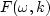can be determined from.
3. What would change in Figure 10 if the pulse were moved (a) earlier on the t-axis, and (b) further on the x-axis? What would change in Figure 10 if instead the time axis were smoothed with (1,4,6,4,1) and the space axis with (1,1)?
4. What would Figure 11 look like on an earth with half the earth velocity?
5. Numerically (or theoretically) compute the two-dimensional spectrum of a plane wave [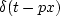], where the plane wave has a randomly fluctuating amplitude: say, rand(x) is a random number between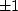,and the randomly modulated plane wave is [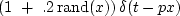].
6. Explain the horizontal layering'' in Figure 9 in the plot of.What determines the layer'' separation? What determines the layer'' slope?Next: HOW FAST FOURIER TRANSFORM Up: TWO-DIMENSIONAL FT Previous: Signs in Fourier transforms
Stanford Exploration Project
10/21/1998